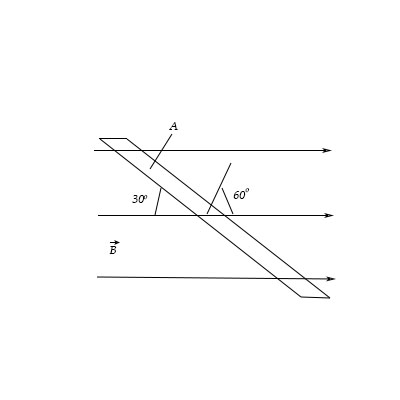# In this example, we use \Phi =BA cos \phi to solve for the strength of magnetic field. A plane...

## Question:

In this example, we use {eq}\Phi =BA \ cos \ \phi {/eq} to solve for the strength of magnetic field. A plane surface with area {eq}4 cm^2 {/eq} is placed in a uniform magnetic field that is oriented at an angle of {eq}30 ^o {/eq} to the surface.

What is the angle {eq}\phi {/eq}? If the magnetic flux through this area is {eq}0.60mWb {/eq}, what is the magnitude of the magnetic field?

For the same {eq}B {/eq} and {eq}A {/eq}, find the angle {eq}\phi {/eq} at which the flux would have one third the value given above (i.e., the angle {eq}\phi {/eq} at which {eq}\Phi_B = 2.8 \times 10^{-4} Wb {/eq}). Express your answer in degrees to two significant figures.## Magnetic Flux:

{eq}\\ {/eq}

The magnetic flux through a surface is the measure of the amount of magnetic field crossing the surface normally.

If a surface is placed in a uniform magnetic field, and surface is a plane, then the magnetic flux through the surface is defined as the product of the area of the surface, and the magnitude of the magnetic field perpendicular to the surface.

If the surface is not plane, or the magnetic field is not uniform, then the magnetic flux is first calculated for an infinitesimal area on the surface. Then this infinitesimal flux is integrated over the entire surface to obtain the total magnetic flux through the surface.

{eq}\\ {/eq}

We are given:

• The area of the plane, {eq}A=4\;\rm cm^2=4\times 10^{-2}\;\rm m^2 {/eq}
• The angle between the magnetic field and the surface, {eq}\theta=30^{\circ} {/eq}
• The magnetic flux through the surface, {eq}\phi=0.6\;\rm mWb=6\times 10^{-4}\;\rm Wb {/eq}

The magnetic flux through a loop of the area, {eq}A {/eq} with a magnetic field, {eq}B {/eq}, is given by the equation:

{eq}\Phi=BA\cos\,\phi {/eq}

Here,

• {eq}\phi {/eq} is the angle between the normal to the loop and the magnetic field.

It is clear from the diagram provided in the question, that the angle between the normal and the magnetic field is:

{eq}\begin{align*} \phi&=90^{\circ}-\theta\\ &=90^{\circ}-30^{\circ}\\ &=60^{\circ} \end{align*} {/eq}

Therefore, according to the question, we have:

{eq}\begin{align*} 6\times 10^{-4}&=B\times 4\times 10^{-4}\times \cos\,60^{\circ}\\ \Rightarrow B&=\dfrac{6}{4\cos\,60^{\circ}}\\ &=\boxed{3\;\rm T} \end{align*} {/eq}

If the magnetic flux decreases to {eq}1/3\rm rd {/eq}, because of the new angle, {eq}\phi' {/eq}, between the magnetic field and the normal, then we have:

{eq}\begin{align*} \Phi'&=\dfrac{\Phi}{3}\\ \Rightarrow BA\cos\,\phi'&=\dfrac{BA\cos\,\phi}{3}\\ \Rightarrow \cos\,\phi&=\dfrac{\cos\,\phi}{3}\\ \Rightarrow \cos\,\phi'&=\dfrac{1}{2\times 3}\\ \Rightarrow \phi'&=\cos^{-1}\,\left ( \dfrac{1}{6} \right )\\ &=\boxed{80.41^{\circ}} \end{align*} {/eq}